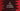# 3 different C programs to print the grade of a student### C program to print the grade of a student:

In this post, we will learn how to print the grade of a student based on the marks obtained. The program will read the marks as input from the user and print the grade for that mark. We will use a predefined ranking system in the program to calculate the grade.

It will use the following grade system:

>=90 A+
>=80 A
>=50 B
>=30 C
<30 F

Based on the value of the marks, the program will print its grade.

### Example 1: C program to print the grade of a student with if-else statements:

Below is the complete C program to print the grade of a student with user-input marks:

``````#include <stdio.h>

int main()
{
int marks;
printf("Enter -1 to exit:\n");
while (1)
{
printf("Enter the mark: ");
scanf("%d", &marks);

if (marks >= 90 && marks <= 100)
{
}
else if (marks >= 80 && marks <= 90)
{
}
else if (marks >= 50 && marks <= 80)
{
}
else if (marks >= 30 && marks <= 50)
{
}
else if (marks >= 0 && marks <= 30)
{
}
else
{
break;
}
}
return 0;
}``````

In this program,

• The while loop runs for an infinite amount of time. It will exit only if the user enters -1.
• It asks the user to enter the value of marks and based on the marks, t prints the grade.
• The if else-if else statements check for the value of the marks and based on its value, it prints the grade. It uses the above table to calculate the grade.
• If the entered value is a negative number, it will break from the condition.

If you run this program, it will print the output as below:

``````Enter -1 to exit:
Enter the mark: 12
Enter the mark: 55
Enter the mark: 90
Enter the mark: 44
Enter the mark: 77
Enter the mark: -1``````

### Example 2: C program to print the grade of a student with a separate function:

We can also use a separate function to write the same program. It will pass the marks to the function and the function will return the grade. Let’s write down the complete program:

``````#include <stdio.h>

{
if (marks >= 90 && marks <= 100)
{
}
else if (marks >= 80 && marks <= 90)
{
}
else if (marks >= 50 && marks <= 80)
{
}
else if (marks >= 30 && marks <= 50)
{
}
else if (marks >= 0 && marks <= 30)
{
}
}
int main()
{
int marks;
printf("Enter -1 to exit:\n");
while (1)
{
printf("Enter the mark: ");
scanf("%d", &marks);

if (marks >= 0)
{
}
else
{
break;
}
}
return 0;
}``````

We created a new function printGrade to print the grades. This function takes the marks as its parameter and it prints the grade. It uses the same if else-if else condition checks as the previous example.

If the value of the user input marks is greater than or equal to zero, it calls this method to print the grade. Else, it breaks from the infinite loop.

It will print similar results.

### Example 3: How to use macro to print the grade of a student:

We can use macros to write this program. It will make the program easy to change. For example, in the above program, if the mark is greater than or equal to 50, it is grade B. If we have to change it to 55, we will have to change this value in two places. If we use macros, it will be defined in one place and the program becomes more readable.

``````#include <stdio.h>
#define MAX 100
#define MIN 0

{
if (marks >= GRADE_A_PLUS_MIN && marks <= MAX)
{
}
{
}
{
}
{
}
else if (marks >= MIN && marks <= GRADE_C_MIN)
{
}
}
int main()
{
int marks;
printf("Enter -1 to exit:\n");
while (1)
{
printf("Enter the mark: ");
scanf("%d", &marks);

if (marks >= 0)
{
}
else
{
break;
}
}
return 0;
}``````

Here,

• We defined a few macros
• MAX is the maximum mark a student can get, i.e. 100 and MIN is the minimum mark i.e. 0.
• GRADE_A_PLUS_MIN is the minimum mark to get Grade A+ i.e. 90.
• GRADE_A_MIN is the minimum mark to get Grade A i.e. 80.
• GRADE_B_MIN is the minimum mark to get Grade B i.e. 50.
• GRADE_C_MIN is the minimum mark to get Grade A i.e. 30.

We replaced the macros in the if else-if else conditions. It will give similar output.